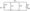# CSVTU Exam Papers – BE I Year – Basic Electrical Engineering – 2005

BE (I  Year)

Examination-2005

Basic Electrical Engineering

Q1 (a)Find current flowing through 5 Ohm resistance of the circuit shown in fig. 1 using The venin’s theorem .(b) Find current through resistance R of the circuit shown in fig. 2 using Nodal voltage method. Take R = 2 Ohm(c) An iron ring of mean length 50cm has an air gap of 1 mm and a winding of 200 turns. If the Relative permeability of iron is 300, find flux density when a current of 1 A flows through winding .

Q2 (a)A resistance R ohms , inductance L=0.01 H capacitance C Farad are connected in series .(Fig. 3) A voltage V given by expression

V= 400 Cos (3000t-10° ) is applied to the circuit , the current flowing is of form i = 10 √2 cos (3000t-55°) find R and C circuit.(b)Find current and power supplied by 200 V source in the circuit shown in fig(c) Three impedance each of value Z= 20600 are connect in star across three phase 400V supply . Find the value current and power supplied what will be these values impedances are connected in delta .

Q3(a)  A single phase transformer has 1000 primary terms and 2 secondary turns . No load current is 2 a at power factor i lag. Secondary load current is 200 A at 0.8 p.f.lag. f current and power factor of primary.

(b) In a 25 KVA single phase transformer iron loss is 350W s full load copper loss is 400 W. Calculate efficiency transformer at fun load cubit power factor and half load puff, lagging .

(c)Write notes on voltage regulation and all day efficiency transformer

Q4(a)) Describe the classification of D.C. motor . Explain the torque speed characteristics

(b) The no load armature current of 23 vole shunt motor is 2 / speed of 1200 RPM . If full load armature current is 40 a full load torque and speed . Take armature resistance = ohm and field flux unchanged form no load to full load .

(c) Explain working principles of three phase induction mc Draw torque step curve for two different values of r resistance .

Q5 (a) (i) What is meant by indicating , recording and integrate instruments give one example of each .

(ii)Explain difference between controlling and dam torque in an electrical instruments

(b) (i) Show a PMMC instruments can be employed as (a) anammeter (b) volt meter.

(ii) How would you extend the range of ammeter  and voltmeter.

(c)With neat diagram explain the construction and| working principles of single phase wattmeter